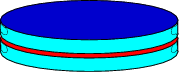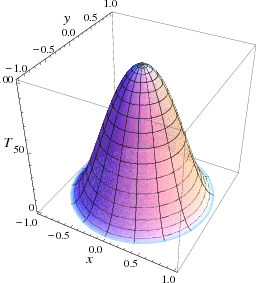$$\newcommand{\id}{\mathrm{id}}$$ $$\newcommand{\Span}{\mathrm{span}}$$ $$\newcommand{\kernel}{\mathrm{null}\,}$$ $$\newcommand{\range}{\mathrm{range}\,}$$ $$\newcommand{\RealPart}{\mathrm{Re}}$$ $$\newcommand{\ImaginaryPart}{\mathrm{Im}}$$ $$\newcommand{\Argument}{\mathrm{Arg}}$$ $$\newcommand{\norm}{\| #1 \|}$$ $$\newcommand{\inner}{\langle #1, #2 \rangle}$$ $$\newcommand{\Span}{\mathrm{span}}$$

# 10.1: Temperature on a Disk

$$\newcommand{\vecs}{\overset { \rightharpoonup} {\mathbf{#1}} }$$ $$\newcommand{\vecd}{\overset{-\!-\!\rightharpoonup}{\vphantom{a}\smash {#1}}}$$$$\newcommand{\id}{\mathrm{id}}$$ $$\newcommand{\Span}{\mathrm{span}}$$ $$\newcommand{\kernel}{\mathrm{null}\,}$$ $$\newcommand{\range}{\mathrm{range}\,}$$ $$\newcommand{\RealPart}{\mathrm{Re}}$$ $$\newcommand{\ImaginaryPart}{\mathrm{Im}}$$ $$\newcommand{\Argument}{\mathrm{Arg}}$$ $$\newcommand{\norm}{\| #1 \|}$$ $$\newcommand{\inner}{\langle #1, #2 \rangle}$$ $$\newcommand{\Span}{\mathrm{span}}$$ $$\newcommand{\id}{\mathrm{id}}$$ $$\newcommand{\Span}{\mathrm{span}}$$ $$\newcommand{\kernel}{\mathrm{null}\,}$$ $$\newcommand{\range}{\mathrm{range}\,}$$ $$\newcommand{\RealPart}{\mathrm{Re}}$$ $$\newcommand{\ImaginaryPart}{\mathrm{Im}}$$ $$\newcommand{\Argument}{\mathrm{Arg}}$$ $$\newcommand{\norm}{\| #1 \|}$$ $$\newcommand{\inner}{\langle #1, #2 \rangle}$$ $$\newcommand{\Span}{\mathrm{span}}$$

Let us now turn to a different two-dimensional problem. A circular disk is prepared in such a way that its initial temperature is radially symmetric, $u(\rho,\phi,t=0) = f(\rho).$ Then it is placed between two perfect insulators and its circumference is connected to a freezer that keeps it at $$0^\circ~\rm C$$, as sketched in Fig. $$\PageIndex{2}$$.Figure $$\PageIndex{1}$$: A circular plate, insulated from above and below.

Since the initial conditions do not depend on $$\phi$$, we expect the solution to be radially symmetric as well, $$u(\rho,t)$$, which satisfies the equation \begin{aligned} \frac{\partial u}{\partial t} &= k\left[\frac{\partial^2 u}{\partial \rho^2} + \frac{1}{\rho} \frac{\partial u}{\partial \rho} \right],\nonumber\\ u(c,t) &=0,\nonumber\\ u(\rho,0) &= f(\rho).\end{aligned}Figure $$\PageIndex{2}$$: The initial temperature in the disk.

Once again we separate variables, $$u(\rho,t)=R(\rho)T(t)$$, which leads to the equation

$\frac{1}{k} \frac{T'}{T} = \frac{R''+\frac{1}{\rho}R'}{R} = -\lambda.$ This corresponds to the two equations

\begin{aligned} \rho^2 R''+\rho R' + \lambda \rho^2 R &= 0,\quad R(c)=0m\nonumber\\ T'+\lambda k T = 0.\end{aligned}

The radial equation (which has a regular singular point at $$\rho=0$$) is closely related to one of the most important equation of mathematical physics, Bessel’s equation. This equation can be reached from the substitution $$\rho = x /\sqrt{\lambda}$$, so that with $$R(r)=X(x)$$ we get the equation

$x^2 \frac{d^2}{dx^2} X(x) + x \frac{d}{dx} X(x) + x^2 X(x) = 0, \qquad X(\sqrt \lambda c) =0.$